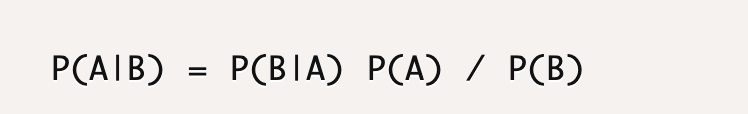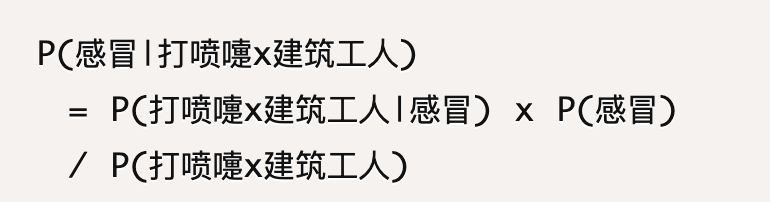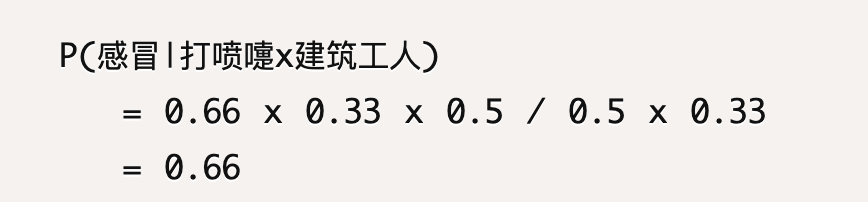## 数据分析经典模型——朴素贝叶斯

6 评论 9631 浏览 57 收藏 9 分钟## 一、条件概率P(white|B)=P(white and B)/P(B)

• P(white and B)=球是白色且球是从B桶中取到的=1/7；
• P(B)=从B桶中取到球的概率=3/7；
• P(white|B)=P(white and B)/P(B)=（1/7）/（3/7）=1/3；

## 二、贝叶斯公式

### 1. 第一步

P(A and B)=P(A|B)*P(B)

2. 第二步

P(A and B)=P(B and A)=P(B|A)*P(A)

### 3. 第三步

P(A|B)=P(A and B)/P(B)

P(A and B)=P(B|A)P(A)

P(A|B)=P(B|A)P(A)/P(B)

• P(A)被称为先验概率；
• P(B|A)被称为后验概率；
• P(B)被称为全概率。

## 三、贝叶斯公式的应用

1. 算出各种不同猜测的可能性大小。
2. 算出最靠谱的猜测是什么。1人打赏1. 如何结合业务使用呢？

回复
2. 让我想起了大学被高数支配的恐惧

回复
3. 有点不太明白最后一个，打喷嚏的建筑工人为什么是0.33*0.5，请赐教，谢谢

回复
1. 感冒是3/6，就是0.5，感冒中有2个打喷嚏，所以是2/3，就是0.66，感冒中有1个建筑工人，所以是1/3，也就是0.33；因为打喷嚏和建筑工人是独立的，所以P(打喷嚏*建筑工人|感冒) = P(打喷嚏|感冒)*P(建筑工人|感冒)

回复
2. 因为打喷嚏和建筑工人是独立的，所以p(打喷嚏*建筑工人)=p(打喷嚏)*p(建筑工人)=3/6*2/6=0.5*0.33

回复
3. 感谢赐教，已懂

回复